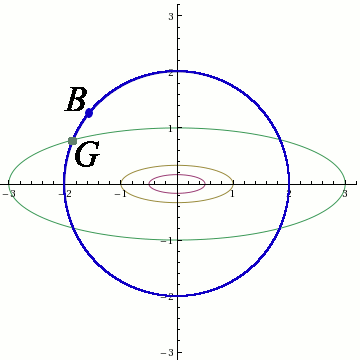# Lagrange Multipliers

## Conditional extremal value problems

Our goal is to solve the problems like this one:

Example 1.

Find the points $$(x,y)$$ on the curve $$x^2+y^2=1$$ for which the quantity $$f(x,y)=xy$$ is maximal.

This problem can be solved using techniques from elementary mathematics, but we’ll resist that temptation. In fact, we’ll use an even simpler example to illustrate the technique of Lagrange multipliers.

Example 2

Find the maximum of the function $$f(x,y)=\frac{x^2}9+y^2$$ if the real numbers $$x$$ and $$y$$ satisfy $$x^2+y^2=4$$.

Our idea is to find the maximum of $$f(x,y)$$ by asking the following sequence of questions:

$$1^{\circ}$$ Is the number 0 the maximum?
$$2^{\circ}$$ Is the number 1 the maximum?
$$3^{\circ}$$ Is the number 0.059 the maximum?
$$4^{\circ}$$ Is the number -11 the maximum?
$$5^{\circ}$$ How about 17?

It seems foolish to hope to answer all these questions. Honestly, it is impossible even to ask all these questions, but let us ignore the reality for a moment. We will answer the above questions by asking easier questions:

$$1^{\circ}$$ Can 0 be a value of the function?
$$2^{\circ}$$ Can 1 be a value of the function?
$$3^{\circ}$$ Can 0.059 be a value of the function?
$$4^{\circ}$$ Can -11 be a value of the function?
$$5^{\circ}$$ Can 17 be a value of the function?

Then we’ll look at all positive answers and circle out the smallest one, and that’s our minimum.

The last set of questions can be rephrased using geometrical language:

$$1^{\circ}$$ Does the level curve $$f(x,y)=0$$ intersect the curve $$x^2+y^2=4$$?
$$2^{\circ}$$ Does the level curve $$f(x,y)=1$$ intersect the curve $$x^2+y^2=4$$?
$$3^{\circ}$$ Does the level curve $$f(x,y)=0.059$$ intersect the curve $$x^2+y^2=4$$?
$$4^{\circ}$$ Does the level curve $$f(x,y)=-17$$ intersect the curve $$x^2+y^2=4$$?
$$5^{\circ}$$ Does the level curve $$f(x,y)=17$$ intersect the curve $$x^2+y^2=4$$?The above picture contains the blue circle $$x^2+y^2=4$$ and several level curves. The level curves are from smallest to largest: $$\frac{x^2}9+y^2=\frac14$$, $$\frac{x^2}9+y^2=1$$, and $$\frac{x^2}9+y^2=3$$. The first two do not intersect the surface hence $$\frac14$$ and $$1$$ are not candidates for the maximum.

Let us analyse the curve $\frac{x^2}9+y^2=3.$ This curve is green and it intersects the blue circle in four points. One of them is prominently displayed with a big green dot and denoted by $$G$$. The value of the function $$f$$ at $$G$$ is equal to $$3$$.

However, this $$G$$ has no chance of being the maximum. There is a blue point $$B$$ that is on the circle and that is outside of this level curve. By being outside of it means that the value of $$f$$ at the point $$B$$ satisfies $$f(B)\neq f(G)$$. There is a point $$B^{\prime}$$ inside the curve $$\frac{x^2}9+y^2=3$$ that also belongs to $$x^2+y^2=4$$ such that $$f(B^{\prime})\neq f(G)$$. We know that one of the numbers $$f(B)$$ and $$f(B^{\prime})$$ is larger than $$f(G)$$ (in this particular example we can prove that it is $$B$$), hence $$G$$ can’t be the maximizer.

We see that the maximizer can be attained only at the points were a level curve is tangent to $$x^2+y^2=4$$.

Two curves are tangent when their normal vectors are parallel. The normal vector of the curve $$f(x,y)=c$$ at the point $$(x_0,y_0)$$ is equal to $$\nabla f(x_0,y_0)$$. Thus the candidates for minima and maxima of $$f$$ are those points of $$x^2+y^2=4$$ for which $$\nabla f\| \langle 2x,2y\rangle$$. Two vectors are parallel if and only if one of them is a multiple of the other. In other words, $$\nabla f\|\langle 2x,2y\rangle$$ if there exists $$\lambda\neq 0$$ such that $$\frac{2x}9=\lambda 2x$$ and $$2u=\lambda 2y$$. In summary, we are looking for the points $$(x,y)$$ that satisfy the following system of equations: $x^2+y^2=4$ $\frac{2x}9=\lambda\cdot 2x$ $2y=\lambda \cdot 2y.$

From the third equation we conclude that $$y=0$$ or $$\lambda = 1$$. In the first case we get $$x=\pm 2$$ and we get two candidates for extrema: $$(2,0)$$ and $$(-2,0)$$.

If $$\lambda =1$$ then the second equation implies that $$x=0$$. From the first one we obtain $$y=\pm 2$$, and the candidates are $$(0,2)$$ and $$(0,-2)$$. Out of these four points we verify that $$f(2,0)=f(-2,0)=\frac49$$ and $$f(0,2)=f(0,-2)=4$$. This means that the maximum of $$f$$ is $$4$$ and is attained for $$(x,y)\in\{(0,2), (0,-2)\}$$. Similarly, the minimum is $$\frac49$$ and is attained for $$(x,y)\in\{(2,0),(-2,0)\}$$.

## The method of Lagrange multipliers

The proof of the following theorem can be completed using the idea of the previous section:

Lagrange multipliers

Assume that $$f(x,y)$$ and $$g(x,y)$$ are continuously differentiable functions. If the maximum or minimum of the function $$f$$ under the condition $$g(x,y)=0$$ occurs at a point $$(x_0,y_0)$$ for which $$\nabla g(x_0,y_0)\neq 0$$, then there exists a constant $$\lambda$$ such that $\nabla f(x_0,y_0)=\lambda \nabla g(x_0,y_0).$

Let us now solve Example 1.

Example 1.

Find the points $$(x,y)$$ on the curve $$x^2+y^2=1$$ for which the quantity $$f(x,y)=xy$$ is maximal.

Example 2.

Let $$S$$ be the surface given by the equation $(2+x-y)^2+(z-x)^2+(z+y)^2=1.$ Find the point on $$S$$ whose distance from the $$z$$-axis is maximal.

2005-2021 IMOmath.com | imomath"at"gmail.com | Math rendered by MathJax